## 4.1.5 The Hydrodynamic Transport Model

The hydrodynamic transport model is again obtained from the BTE by the moment methods, however it accounts for the first three moments instead of only two as the DD transport model. The resulting equations are: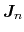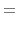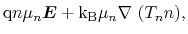(4.16)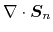(4.17)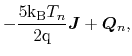(4.18)

for electrons, where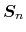is the energy heat flux,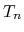is the electron temperature,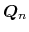is the heat flow, and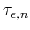is the energy relaxation time of the electrons. The model is closed by using Fourier's law for the heat term: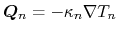(4.19)

where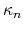is the thermal conductivity. The latter is calculated by the Wiedemann-Franz law, proportional to the mobility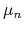and carrier temperature: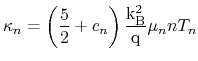(4.20)

The factor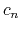(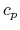for holes, respectively) is set to zero so that both terms of (4.18) have the same factor and any inconsistency can be avoided. The model obtained is actually an energy transport model as the moment equations were derived under the assumption that the average kinetic energy of the carriers is negligible in comparison to their thermal energy: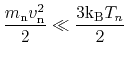(4.21)

However, since in literature the terms energy transport and hydrodynamic transport are often used interchangeably, the name hydrodynamic transport is further retained.

S. Vitanov: Simulation of High Electron Mobility Transistors